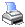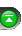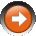﻿ Standard Error (StdError)
 NinjaScript > Language Reference > Indicator Methods > Standard Error (StdError)Description

The standard error of a method of measurement or estimation is the standard deviation of the sampling distribution associated with the estimation method. The term may also be used to refer to an estimate of that standard deviation, derived from a particular sample used to compute the estimate.

... Courtesy of Wikipedia

Syntax

 StdError(int period) StdError(IDataSeries input, int period)   Returns default value which is the mid line (also known as linear regression) StdError(int period)[int barsAgo] StdError(IDataSeries input, int period)[int barsAgo]   Returns upper value StdError(int period).Upper[int barsAgo] StdError(IDataSeries input, int period).Upper[int barsAgo]   Returns lower value StdError(int period).Lower[int barsAgo] StdError(IDataSeries input, int period).Lower[int barsAgo]

Return Value

double; Accessing this method via an index value [int barsAgo] returns the indicator value of the referenced bar.

Parameters

 input Indicator source data (?) period Number of bars used in the calculation

Examples

 // Prints the current upper value of a 20 period StdError using default price type double value = StdError(20).Upper; Print("The current upper Standard Error value is " + value.ToString());

Source Code

You can view this indicator method source code by selecting the menu Tools > Edit NinjaScript > Indicator within the NinjaTrader Control Center window.